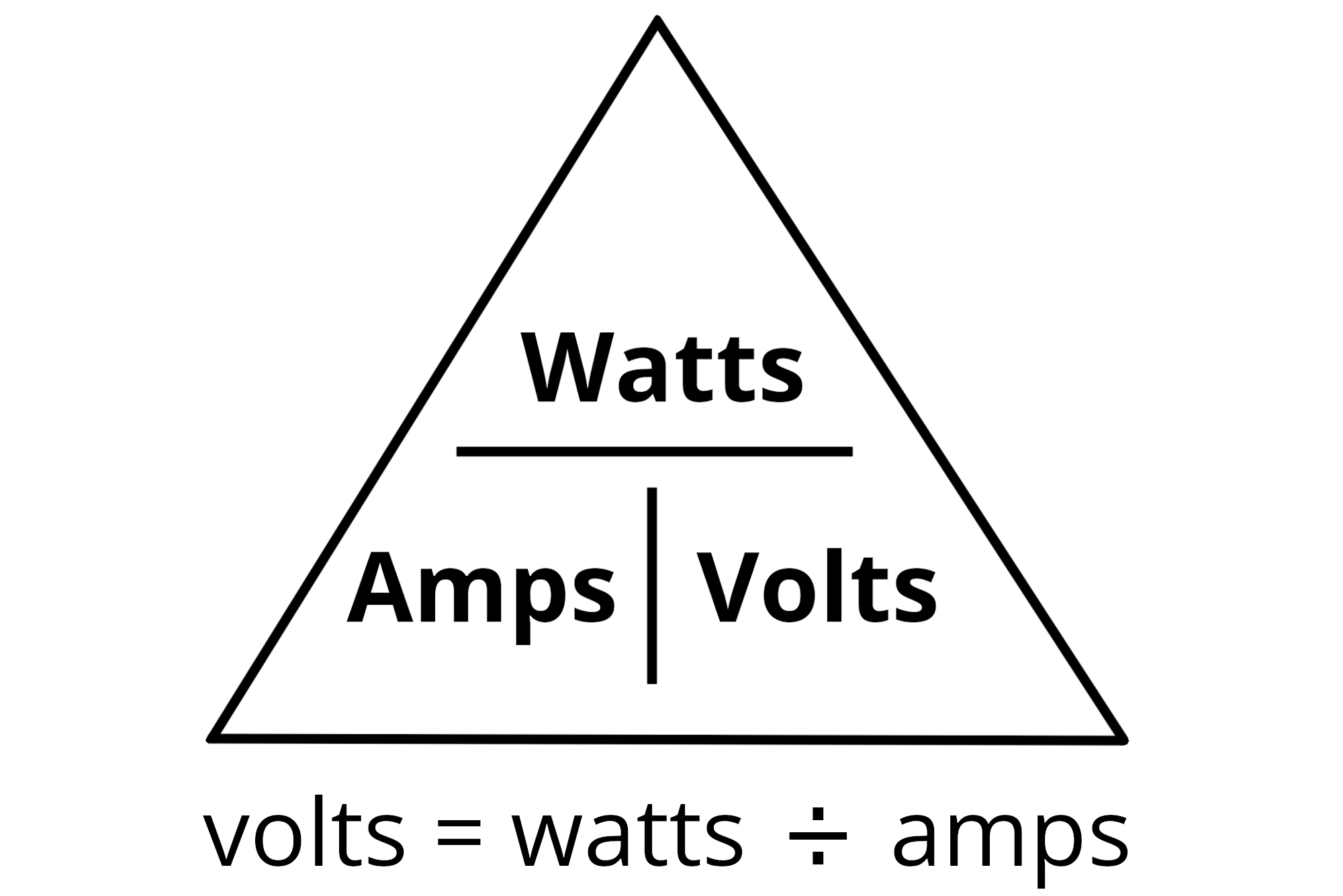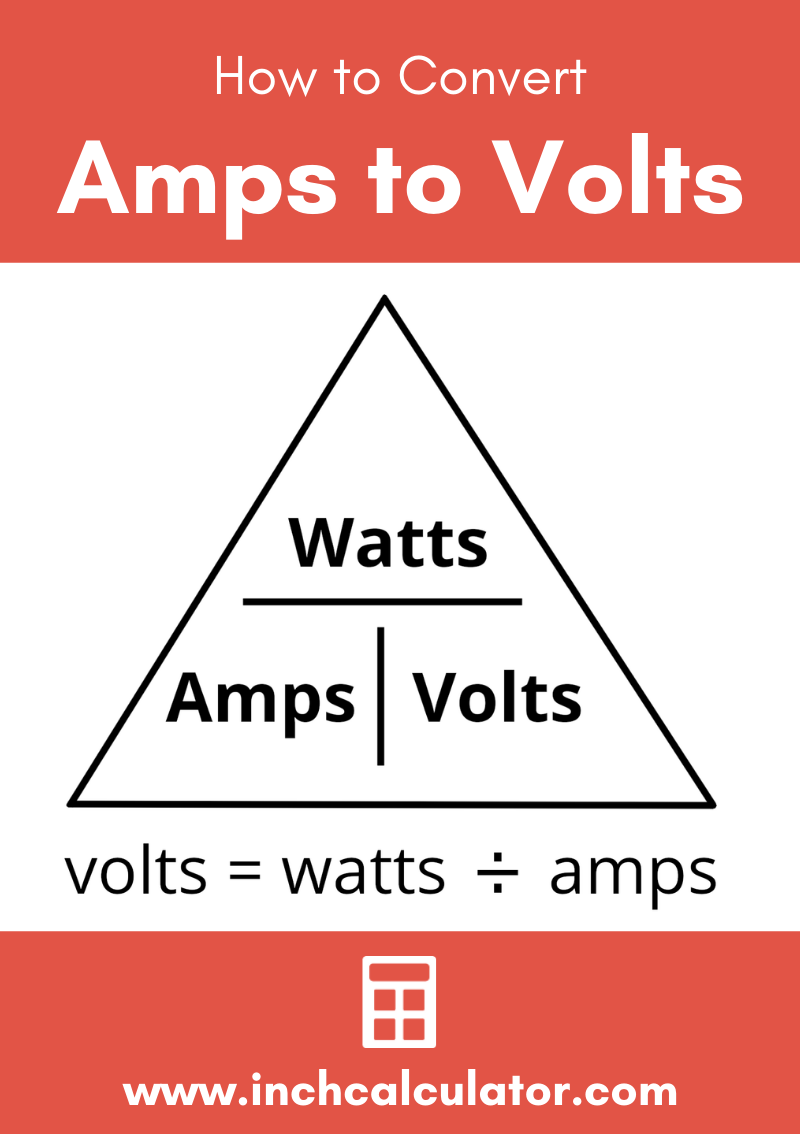# Amps to Volts Conversion Calculator

Convert amps to volts to find the voltage across the terminals of an electrical device in volts. Enter the current in amps and the power in watts or the amps and the resistance in ohms below to calculate the voltage.

## Results:

Volts
Learn how we calculated this below

## How to Convert Amps to Volts

Amps, short for amperes, are a standard unit to measure the electrical current flowing in an electrical circuit. A current of 1 A is equivalent to a rate of flow of charge of 1 Coulomb in 1 second.

Voltage is the measure of the electric potential in an electric circuit to move an electric charge. It is measured in Volts; 1 Volt equals the potential needed to move 1 A of current against a 1 ohm of resistance.

Two methods are generally used to convert Amps to Volts, the Watt’s Law power formula and the Ohm’s Law formula.

### Conversion Using the Power Formula

To convert amps to volts, we can use the power formula that allows us to derive the equation for calculating the voltage. Per the power formula, voltage is equal to the power delivered to a device divided by the current that flows through it:

V(V) = P(W) ÷ I(A)

The voltage V is equal to the power P in watts divided by the current I in amps.For example, let’s find the voltage of a device that uses 120 watts of power with a current of 10 amps.

V(V) = 120 W ÷ 10 A
V(V) = 12 V

### Conversion Using Ohm’s Law

It is also possible to convert amps to volts if the resistance is known, thanks to the Ohm’s Law formula. Using Ohm’s Law, we can state that voltage is equal to electrical current times the resistance.

V(V) = I(A) × R(Ω)

The voltage V is equal to the current I in amps times the resistance R in ohms.

For example, let’s find the voltage of a circuit with 1.2 amps of current and 20 ohms of resistance.

V(V) = 1.2 A ÷ 20 Ω
V(V) = 24 V

## Amps to Volts Conversion Table

Amperes to voltage conversions based on the power formula.

Table showing amps converted to volts at various power ratings.
Current (A) Voltage (V) Power (W)
1 amp 5 volts 5 watts
1 amp 10 volts 10 watts
1 amp 15 volts 15 watts
1 amp 20 volts 20 watts
1 amp 25 volts 25 watts
1 amp 30 volts 30 watts
1 amp 35 volts 35 watts
1 amp 40 volts 40 watts
1 amp 45 volts 45 watts
1 amp 50 volts 50 watts
1 amp 55 volts 55 watts
1 amp 60 volts 60 watts
1 amp 65 volts 65 watts
1 amp 70 volts 70 watts
1 amp 75 volts 75 watts
1 amp 80 volts 80 watts
1 amp 85 volts 85 watts
1 amp 90 volts 90 watts
1 amp 95 volts 95 watts
1 amp 100 volts 100 watts
2 amps 2.5 volts 5 watts
2 amps 5 volts 10 watts
2 amps 7.5 volts 15 watts
2 amps 10 volts 20 watts
2 amps 12.5 volts 25 watts
2 amps 15 volts 30 watts
2 amps 17.5 volts 35 watts
2 amps 20 volts 40 watts
2 amps 22.5 volts 45 watts
2 amps 25 volts 50 watts
2 amps 27.5 volts 55 watts
2 amps 30 volts 60 watts
2 amps 32.5 volts 65 watts
2 amps 35 volts 70 watts
2 amps 37.5 volts 75 watts
2 amps 40 volts 80 watts
2 amps 42.5 volts 85 watts
2 amps 45 volts 90 watts
2 amps 47.5 volts 95 watts
2 amps 50 volts 100 watts
3 amps 1.667 volts 5 watts
3 amps 3.333 volts 10 watts
3 amps 5 volts 15 watts
3 amps 6.667 volts 20 watts
3 amps 8.333 volts 25 watts
3 amps 10 volts 30 watts
3 amps 11.667 volts 35 watts
3 amps 13.333 volts 40 watts
3 amps 15 volts 45 watts
3 amps 16.667 volts 50 watts
3 amps 18.333 volts 55 watts
3 amps 20 volts 60 watts
3 amps 21.667 volts 65 watts
3 amps 23.333 volts 70 watts
3 amps 25 volts 75 watts
3 amps 26.667 volts 80 watts
3 amps 28.333 volts 85 watts
3 amps 30 volts 90 watts
3 amps 31.667 volts 95 watts
3 amps 33.333 volts 100 watts
4 amps 1.25 volts 5 watts
4 amps 2.5 volts 10 watts
4 amps 3.75 volts 15 watts
4 amps 5 volts 20 watts
4 amps 6.25 volts 25 watts
4 amps 7.5 volts 30 watts
4 amps 8.75 volts 35 watts
4 amps 10 volts 40 watts
4 amps 11.25 volts 45 watts
4 amps 12.5 volts 50 watts
4 amps 13.75 volts 55 watts
4 amps 15 volts 60 watts
4 amps 16.25 volts 65 watts
4 amps 17.5 volts 70 watts
4 amps 18.75 volts 75 watts
4 amps 20 volts 80 watts
4 amps 21.25 volts 85 watts
4 amps 22.5 volts 90 watts
4 amps 23.75 volts 95 watts
4 amps 25 volts 100 watts